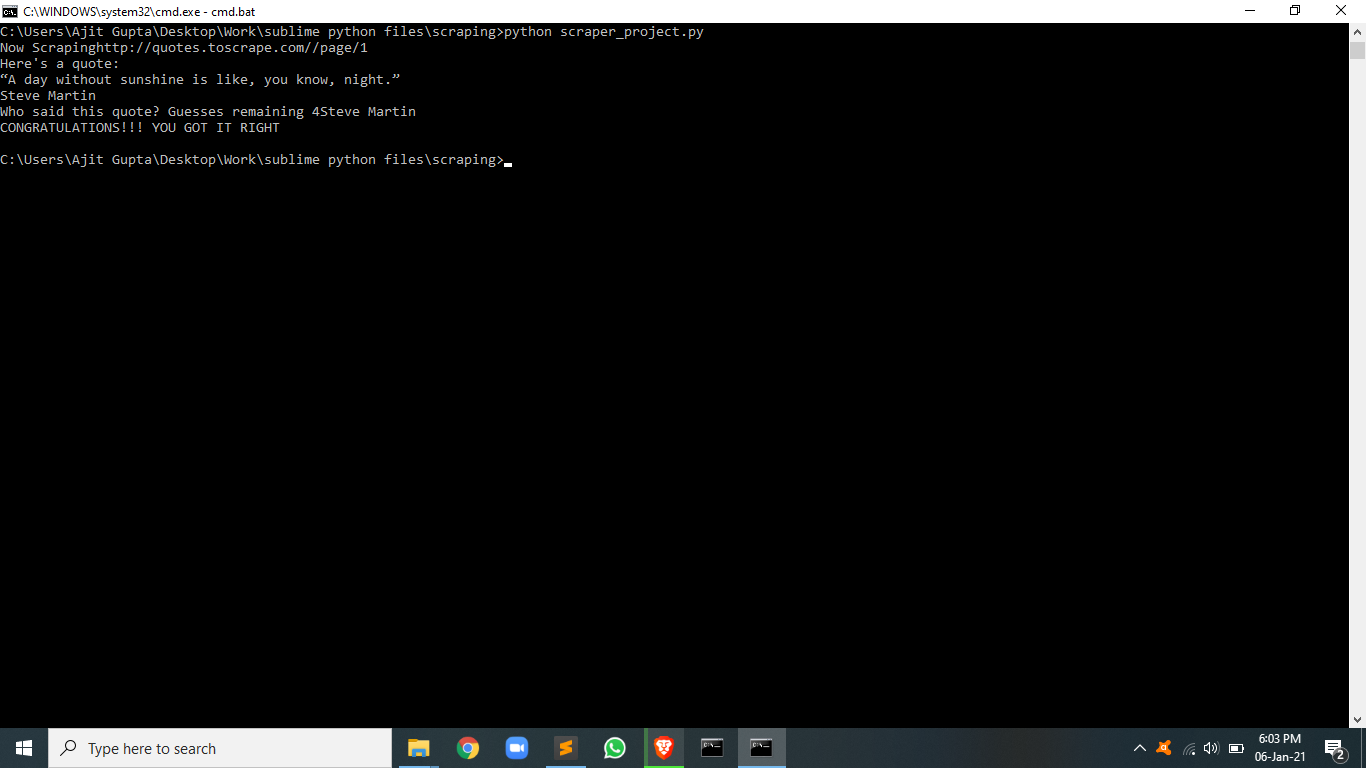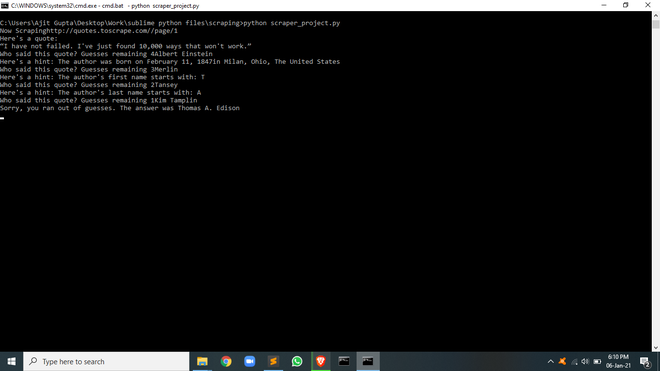Open in App
Not now

# Quote Guessing Game using Web Scraping in Python

• Difficulty Level : Medium
• Last Updated : 27 Jan, 2023

Prerequisite: BeautifulSoup Installation

In this article, we will scrape a quote and details of the author from this site http//quotes.toscrape.com using python framework called BeautifulSoup and develop a guessing game using different data structures and algorithm.

The user will be given 4 chances to guess the author of a famous quote, In every chance the user will be provided with a hint which can be the author’s birth date, first name’s first letter, second name’s first letter, etc. On successfully guessing the author, a message is printed and if the user fails to guess the answer even after all the 4 chances then again a message is printed along with the answer.

### Approach

• Import module
• requests help us grab the page, when the response is received it is stored in the form of a string
• bs4 library is used to create beasutifulSoup object.
• csv library helps reading and writing CSV files using python
• sleep function from time module helps add delay in the execution of the program.
• choice function from random module returns a random element.
• Create a list to store values scraped
• Scrape the details from this link: http//quotes.toscrape.com
• Extract data
• Game logic
• Return random items from the dictionary created
• Set number of guesses
• Write message for success and failure
• Keep giving hints until either number of chances reach zero or the user gets it right

Program:

## Python3

 `import` `requests` `from` `bs4 ``import` `BeautifulSoup` `from` `csv ``import` `writer` `from` `time ``import` `sleep` `from` `random ``import` `choice`   `# list to store scraped data` `all_quotes ``=` `[]`   `# this part of the url is constant` `base_url ``=` `"http://quotes.toscrape.com/"`   `# this part of the url will keep changing` `url ``=` `"/page/1"`   `while` `url:` `  `  `    ``# concatenating both urls` `    ``# making request` `    ``res ``=` `requests.get(f``"{base_url}{url}"``)` `    ``print``(f``"Now Scraping{base_url}{url}"``)` `    ``soup ``=` `BeautifulSoup(res.text, ``"html.parser"``)`   `    ``# extracting all elements` `    ``quotes ``=` `soup.find_all(``class_``=``"quote"``)`   `    ``for` `quote ``in` `quotes:` `        ``all_quotes.append({` `            ``"text"``: quote.find(``class_``=``"text"``).get_text(),` `            ``"author"``: quote.find(``class_``=``"author"``).get_text(),` `            ``"bio-link"``: quote.find(``"a"``)[``"href"``]` `        ``})` `    ``next_btn ``=` `soup.find(_class``=``"next"``)` `    ``url ``=` `next_btn.find(``"a"``)[``"href"``] ``if` `next_btn ``else` `None` `    ``sleep(``2``)`   `quote ``=` `choice(all_quotes)` `remaining_guesses ``=` `4` `print``(``"Here's a quote:  "``)` `print``(quote[``"text"``])`   `guess ``=` `''` `while` `guess.lower() !``=` `quote[``"author"``].lower() ``and` `remaining_guesses > ``0``:` `    ``guess ``=` `input``(` `        ``f``"Who said this quote? Guesses remaining {remaining_guesses}"``)` `    `  `    ``if` `guess ``=``=` `quote[``"author"``]:` `        ``print``(``"CONGRATULATIONS!!! YOU GOT IT RIGHT"``)` `        ``break` `    ``remaining_guesses ``-``=` `1` `    `  `    ``if` `remaining_guesses ``=``=` `3``:` `        ``res ``=` `requests.get(f``"{base_url}{quote['bio-link']}"``)` `        ``soup ``=` `BeautifulSoup(res.text, ``"html.parser"``)` `        ``birth_date ``=` `soup.find(``class_``=``"author-born-date"``).get_text()` `        ``birth_place ``=` `soup.find(``class_``=``"author-born-location"``).get_text()` `        ``print``(` `            ``f``"Here's a hint: The author was born on {birth_date}{birth_place}"``)` `    `  `    ``elif` `remaining_guesses ``=``=` `2``:` `        ``print``(` `            ``f``"Here's a hint: The author's first name starts with: {quote['author']}"``)` `    `  `    ``elif` `remaining_guesses ``=``=` `1``:` `        ``last_initial ``=` `quote[``"author"``].split(``" "``)[``1``][``0``]` `        ``print``(` `            ``f``"Here's a hint: The author's last name starts with: {last_initial}"``)` `    `  `    ``else``:` `        ``print``(` `            ``f``"Sorry, you ran out of guesses. The answer was {quote['author']}"``)`

Output:Time Complexity:
The time complexity of this code is O(N) because the while loop is iterating over quotes list N times.

Space Complexity:
The space complexity of this code is O(N) because the all_quotes list is storing N number of elements.

My Personal Notes arrow_drop_up
Related Articles